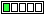All about flooble | fun stuff | Get a free chatterbox | Free JavaScript | Avatarsperplexus dot infoA quicky (Posted on 2012-07-31)Find the smallest multiple of 9 with no odd digits.

Use your head ONLY.

 See The Solution Submitted by Ady TZIDON Rating: 5.0000 (2 votes)Comments: ( Back to comment list | You must be logged in to post comments.)Solution| Comment 1 of 6
288

Explanation:

At the outset, we shall assume non leading zeroes.

The sum of digits of the number must be 9.

The only single digit number which is a multiple of 9 is 9, which is odd and accordingly untenable.

If it is a two digit number, then the sum of digits must be at least 9 and at most 18. If the sum of digits is 9, then one digit is odd and the other even.
If the sum of digits is 18, then the number must be 99. Thus, it is not possible for the number to have two digits.

If the number has three digits, the possible sum of digits are:9, 18 and 27.

But, for the s.s.d to be 9, two even digits or, two odd digits will yield the remaining digit as odd. If the s.s.d is 18, then the minimum digit in the hundreds place must be 2. So the sum of the digits in the tens and the units place is 16. Since all the digits must be even, the minimum is obtained whenever, each of the digit in the tens place and the digit in the units place is 8.

Accordingly, the minimum base ten positive integer satisfying the given conditions is 288.

------------------------------------------------------------------------------

Edited on July 31, 2012, 4:01 pm
 Posted by K Sengupta on 2012-07-31 15:55:48Please log in:
 Login: Password: Remember me: Sign up! | Forgot password

 Search: Search body:
Forums (0)
Newest Problems
Random Problem
FAQ | About This Site
Site Statistics
New Comments (2)
Unsolved Problems
Top Rated Problems
This month's top
Most Commented On

Chatterbox:
Copyright © 2002 - 2021 by Animus Pactum Consulting. All rights reserved. Privacy Information